Next: Synthetics Up: Model fitting by least Previous: INVERSE NMO STACK

# MARINE DEGHOSTING

The marine ghost presents a problem that is essentially insoluble; but because it is always with us, we need to understand how to do the best we can with it. Even if an airgun could emit a perfect impulse, the impulse would reflect from the nearby water surface, thereby giving a second pulse of opposite polarity. The energy going down into the earth is therefore a doublet when we would prefer a single pulse. Likewise, hydrophones see the upcoming wave once coming up, and an instant later they see the wave with opposite polarity reflecting from the water surface. Thus the combined system is effectively a second derivative wavelet (1,-2,1) that is convolved with signals of interest. Our problem is to remove this wavelet by deconvolution. It is an omnipresent problem and is cleanly exposed on marine data where the water bottom is hard and deep.

Theoretically, a double integration of the second derivative gives the desired pulse. A representation in the discrete time domain is the product of (1-Z)2 with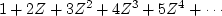, which is 1. Double integration amounts to spectral division by.Mathematically the problem is thatvanishes at.In practice the problem is that dividing by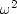where it is small amplifies noises at those low frequencies. (Inversion theorists are even more frustrated because they are trying to create something like a velocity profile, roughly a step function, and they need to do something like a third integration.) Old nuts like this illustrate the dichotomy between theory and practice.

Chapterprovides a theoretical solution to this problem in the Fourier domain. Here we will express the same concepts in the time domain. Define as follows:


yt 		 Given data.
bt 		 Known filter.
xt 		 Excitation (to be found).Noise: data minus filtered excitation.

With Z-transforms the problem is given by Y(Z)=B(Z)X(Z)+N(Z). Our primary wish is.Our secondary wish is that X not be infinity as X=Y/B threatens. This second wish is expressed as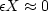and is called stabilizing" or damping." In the Fourier domain the wishes are(50) (51)
The formal expression of the regression is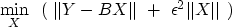(52)
In the time domain the regression is much more explicit: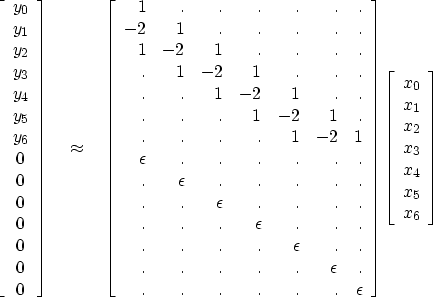(53)
where'' denotes a zero. Since it is common to addto an operator to stabilize it, I prepared subroutine ident() for this purpose. It is used so frequently that I coded it in a special way to allow the input and output to overlie one another.

subroutine ident( adj, add, epsilon, n, pp,    qq   )
real                        epsilon,    pp(n), qq(n)  # equivalence (pp,qq) OK
if( adj == 0 ) {
if( add == 0 ) { do i=1,n {  qq(i) =         epsilon * pp(i) } }
else           { do i=1,n {  qq(i) = qq(i) + epsilon * pp(i) } }
}
else {  if( add == 0 ) { do i=1,n {  pp(i) =         epsilon * qq(i) } }
else           { do i=1,n {  pp(i) = pp(i) + epsilon * qq(i) } }
}
return; end


We can use any convolution routine we like, but for simplicity, I selected contrunc() so the output would be the same length as the input. The two operators ident() and contrunc() could be built into a new operator. I found it easier to simply cascade them in the deghosting subroutine deghost() below.

# deghost:                   min       |rrtop|  =  | y - bb (contrunc) xx |
#                             x        |rrbot|     | 0 - epsilon  I    xx |
subroutine deghost( eps, nb,bb, n, yy, xx, rr, niter)
integer iter,            nb,    n,             niter
real    bb(nb), yy(n), eps              # inputs.  typically bb=(1,-2,1)
real    xx(n), rr(n+n)                  # outputs.
temporary real dx(n), sx(n), dr(n+n), sr(n+n)
call zero( n, xx)
call copy( n, yy, rr(1  ))        # top half of residual
call zero( n    , rr(1+n))        # bottom   of residual
do iter= 0, niter {
call contrunc(1,0,1,nb,bb, n,dx,n,rr); call ident(1,1,eps, n,dx,rr(1+n))
call contrunc(0,0,1,nb,bb, n,dx,n,dr); call ident(0,0,eps, n,dx,dr(1+n))
call cgstep( iter,  n,xx,dx,sx, _
n+n,rr,dr,sr)
}
return; endNext: Synthetics Up: Model fitting by least Previous: INVERSE NMO STACK
Stanford Exploration Project
10/21/1998